• Call Now

1800-102-2727•

# Modulus Of Elasticity- Young’s Modulus, Formula, Practice Problems, FAQs

If you have two rods made up of two different materials and someone asked you that, which rod is having more strength? So to come to the conclusion you will generate stress in the rod by applying some force. Then you will check which rod is able to withstand higher stress. What if I tell you that there is a well defined quantity that tells us exactly about the strength of material. This property is nothing but modulus of elasticity. In this article we will study about Young's modulus of elasticity in detail.

• Modulus of elasticity
• Unit and dimension of modulus of elasticity
• Young’s modulus of elasticity
• Elongation in rod due to self-weight
• Factors Affecting Elasticity
• Properties of Young’s Modulus (Y)
• Value of Young’s Modulus for Different Materials
• Practice problems
• FAQs

## Modulus of Elasticity

According to Hooke’s law, within proportional limit the stress produced in material is proportional to the strain. The constant of proportionality is called modulus of elasticity.

So mathematically,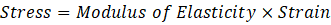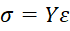Formula for Modulus of elasticity,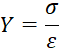Here,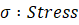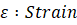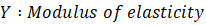Corresponding to three types of strain (longitudinal, shear and volumetric) there are three types of moduli of elasticity accompanied with each type of strain as follows:

• Young’s modulus of elasticity (Y)
• Modulus of rigidity (η)
• Bulk modulus of elasticity (B)

In this article we will be mainly focusing on Young’s modulus of elasticity.

## Unit and Dimension of Modulus of Elasticity

Modulus of elasticity has the same unit and dimension as that of stress.

SI Unit :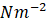Dimension: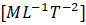## Young’s Modulus of Elasticity

It is defined as the ratio of the normal stress to the longitudinal strain.

Normal stress or longitudinal stress is defined as,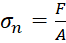Longitudinal strain is defined as,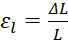Hence, Young’s modulus of elasticity is written as,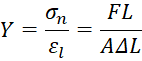It is predominantly used only for solids.

Note:

• Extension (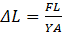) is proportional to the deforming force and length of rod, i.e. greater the force larger the deformation and longer the rod larger the elongation.
• Extension is inversely proportional to the cross sectional area and modulus of elasticity, i.e. a thicker rod produces less deformation and a stiffer material produces less elongation.

## Elongation In Rod Due To Self-Weight

Consider a rod having length L, weight W, area of cross section as A, and Young’s modulus of elasticity as Y. Different parts of the rod do not elongate to the same extent. The elements closer to the support elongate more as the stress is higher with respect to the elements closer to the free end of the rod.

Consider a thin, disc-shaped element of thickness dx at distance x from the lower end of the rod.The net force on the element is the following: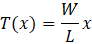Here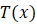means that T is dependent on x.

We know,So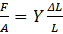For small element dx, consider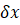elongation

Thus for small element above equation can be written as,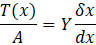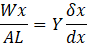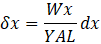Integrate on both side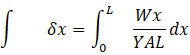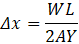Hence, the net elongation in the rod due to its self-weight is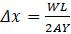.

## Factors Affecting Elasticity

• Effect of Temperature: Almost for all materials, the modulus of elasticity decreases with increase in temperature but the elasticity of invar remains constant with rise in temperature.
• Effect of Impurities: The addition of impurities affects the elastic properties depending on whether impurities are themselves more or less elastic.
• Effect of Annealing: By annealing large crystal grains are formed and hence the elasticity of material decreases.
• Effect of Hammering and Rolling: By hammering and rolling, crystal grains break into smaller units and hence the elasticity of material increases.

## Properties of Young’s Modulus (Y)

• Value of Y depends only on the nature of the material.
• Larger the value of Y for a material, the more elastic it would be.
• Young’s modulus is only defined for solids not for liquids and gases.
• Young’s modulus for a perfectly rigid body is infinite.
• Breaking stress does not depend on the cross sectional area. It only depends on the material of the wire.

Value of Young’s Modulus for Different Materials

## Practice Problems

Q. A system of three massless rods of area of cross section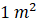is given in the figure. A block of mass 10 kg is attached at the end of the lowest rod. Find the shift in points B after the mass is attached?[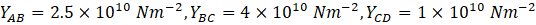and length of rod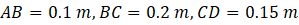]

A. The given three rods are massless and the applied force on each rod will be equal in magnitude.

Area of cross section (A) of the given three rods is unity (i.e.) and gravitational acceleration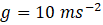Therefore, the force on each rod (AB, BC, and CD) is,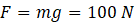Now,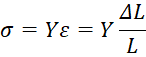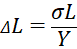Therefore, the change in the length of rod AB is as follows: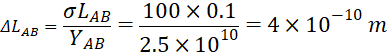The shift in point B is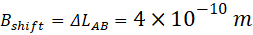Q. A system of three massless rods of area of cross sectionis given in the figure. A block of mass 10 kg is attached at the end of the lowest rod. Find the shift in points D after the mass is attached?[and length of rod]

A. The given three rods are massless and the applied force on each rod will be equal in magnitude.

Area of cross section (A) of the given three rods is unity (i.e.) and gravitational accelerationTherefore, the force on each rod (AB, BC, and CD) is,Now,Therefore, the change in the length of rod AB is as follows:The change in the length of rod BC is as follows: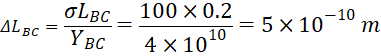The change in the length of rod CD is as follows: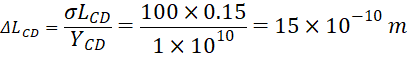The shift in point D is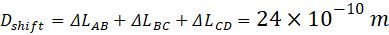Q. A load of 10 KN is supported from a pulley which in turn is supported by a rope of sectional area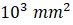and modulus of elasticity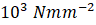, as shown in the figure. Neglecting the friction at the pulley, determine the deflection of the load?A. Let T be the tension in the rope. Then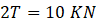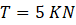Hence the longitudinal stress in the rope is,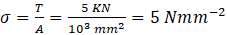Total Extension in the rope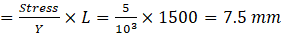Therefore the deflection of the load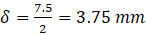Q. A uniform rod of mass m and length L is pulled horizontally on a smooth surface with a force F. Determine the elongation of the rod if Young’s modulus of elasticity of the material is Y? [Area of cross section is A]A. Under the action of the external force, the rod accelerates with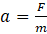. The magnitude of stress decreases as one moves away from the point of application of the force. Therefore, the strain also decreases as one moves towards the free end.

To determine the total elongation of the rod, let us consider a small element of length dx at a distance x from the free end of the rod. The magnitude of force at this section is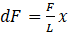.

The stress at this section is,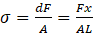The elongation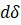produced in this differential element is,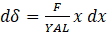Thus, total elongation is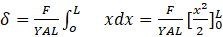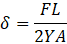## FAQs

Q. What will be the Young’s modulus of a perfectly rigid body?

A. In a perfectly rigid body there will not be any deformation after the application of force. So the Young;s modulus will be infinite.

Q. If we increase the stress on the wire, the ratio of stress and strain will?

A. The ratio of stress and strain is modulus of elasticity and it is the property of material. So even if we increase the stress the ratio of stress to strain will remain the same.

Q. What affects the elasticity of a material?

A. Impurity in the material, temperature, hammering and annealing affects the elasticity.

Q. How can we compare the strength of two materials?

A. By comparing the Young’s modulus of elasticity we can compare the strength of materials. Higher the Young’s modulus higher the strength of material.

Q. What is more elastic, iron rod or rubber band?

A. An iron rod is more elastic than a rubber band. As iron rod has a very high value of Y from rubber.Talk to our expert
Resend OTP Timer =
By submitting up, I agree to receive all the Whatsapp communication on my registered number and Aakash terms and conditions and privacy policy